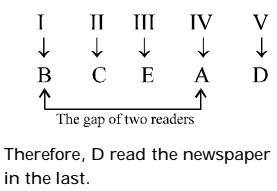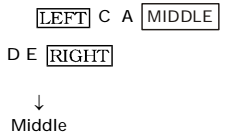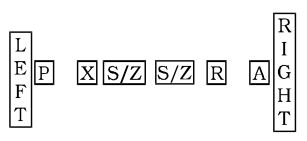## Sitting Arrangement

#### Sitting Arrangement

1. There are five different houses, A to E, in a row. A is to the right of B and E is to the left of C and right of A, B is to the right of D. Which of the houses is in the middle ?

1. According to question
A is to the right of B :: then B   A
E is to the left of C and right of A :: then A   E   C
B is to the right of D :: then D   B

Data is arranged as
D B A E C
.   .   .   .  .

##### Correct Option: A

According to question
A is to the right of B :: then B   A
E is to the left of C and right of A :: then A   E   C
B is to the right of D :: then D   B

Data is arranged as
D B A E C
.   .   .   .  .
So,the house in the middle is A

1. A, B, C, D, E and F are sitting in a row. 'E' and 'F' are in the center and 'A' and 'B' are at the ends. 'C' is sitting on the left of 'A'. Then who is sitting on the right of 'B' ?

1. According to the question, setting arrangement is at follow
'A' and 'B' are at the end then
B           A
'C' is sitting on the left of 'A' then
B       C   A
'E' and 'F' are in the center then arrangement will be
B D   E/F   F/E    C A
.   .     .     .  .

##### Correct Option: B

According to the question, setting arrangement is at follow
'A' and 'B' are at the end then
B           A
'C' is sitting on the left of 'A' then
B       C   A
'E' and 'F' are in the center then arrangement will be
B D   E/F   F/E    C A
.   .     .     .  .
Clearly, D it sitting on the right of B.

1. 5 men A, B, C, D and E read a newspaper. The one who reads first gives it to C, the one who reads last had taken it from A. E was not the first or last to read. There were two readers between B and A. Find the person who read the newspaper last.

1. According to question,
C is 2nd person who read the news paper. Keep him at 2nd position.
E was not the first or last to read. It means E will seat at 3rd or 4th position.
................... Arrange the queue in same pattern as per given question.##### Correct Option: C

According to question,
C is 2nd person who read the news paper. Keep him at 2nd position.
E was not the first or last to read. It means E will seat at 3rd or 4th position.
There were two readers between B and A. Then definitely C and E will seat between B and A. So E will be at 3rd position since B is already at 2nd position.
The one who reads last had taken it from A. Then A will be at 4th position.
Finally D will be at 5th position who will read the news in last.1. Of the 5 members of a panel sitting in a row, A is to the left of B, but on the right of C, D is on the right of B but is on the left of E. Find the member who is sitting in the middle.

1. According to question,
A is to the left of B, but on the right of C, D is on the right of B but is on the left of E.##### Correct Option: A

According to question,
Draw a figure A is to the left of B, but on the right of C, D is on the right of B but is on the left of E.1. A, P, R, X, S and Z are sitting in a row. S and Z are in the centre, and A and P are at the ends. R is sitting on the left of A. Then who is on the right of P?

1. According to question,
Draw a figure S and Z are in the centre, and A and P are at the ends. R is sitting on the left of A.##### Correct Option: B

According to question,
Draw a figure S and Z are in the centre, and A and P are at the ends. R is sitting on the left of A. Hence, X is on the right of P.